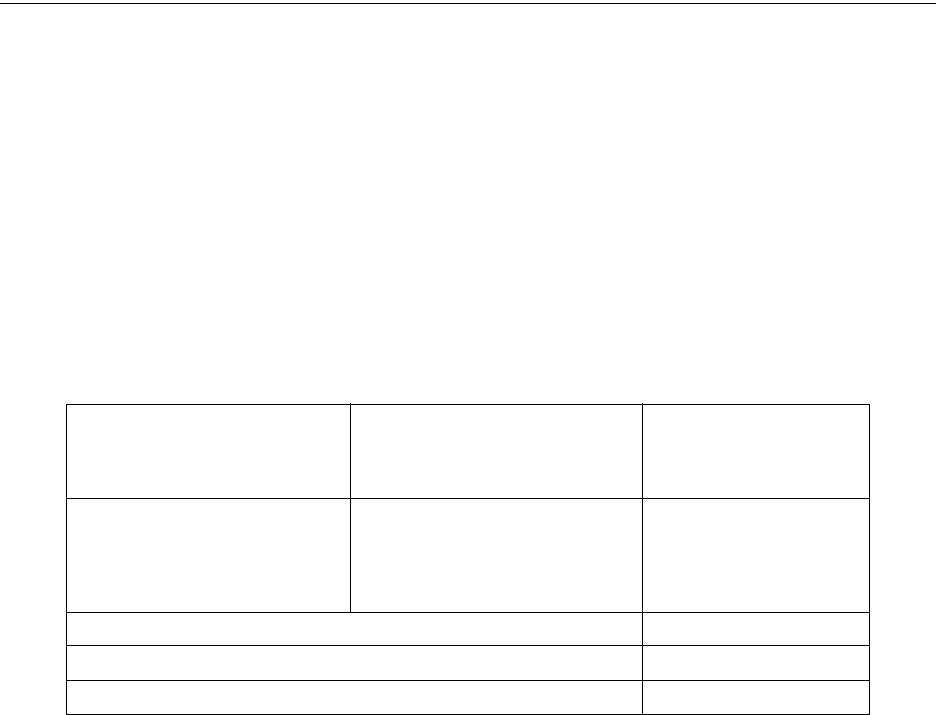# User guide74 Distributed Audio Panel Manual — P/N 52265:B1 6/8/2010
Power Supply Calculations Calculating the Battery Size
6.4 Calculating the Battery Size
Use Table 6.3 to calculate the total Standby and Alarm load in ampere hours (AH). This total load
determines the battery size (in AH), required to support the ACC-25/50DA under the loss of AC
power. Complete Table 6.3 as follows:
1. Enter the totals from Table 6.2 on page 73 Calculation Columns 1 and 2 where shown.
2. Enter the NFPA Standby and Alarm times (refer to Section 6.4.1, 'NFPA Battery
Requirements').
3. Calculate the ampere hours for Standby and Alarm, then sum the Standby and Alarm ampere
hours.
4. Multiply the sum by the derating factor of 1.2 to get the proper battery size (in AH).
5. Write the ampere hour requirements on the Protected Premises label located inside the cabinet
door.
6.4.1 NFPA Battery Requirements
NFPA requires 24 hours of standby plus 15 minutes activation for audio systems. The total ampere
hours required cannot exceed 18 AH with an internal charger. An external charger can be used to
increase the total ampere hours (internal charger must be disabled).
6.4.2 Selecting and Locating Batteries
Select batteries that meet or exceed the total ampere hours calculated in Table 6.3. The audio panel
can charge batteries in the 7 AH to 18 AH range. The ACC-25/50DA Series can house up to 18 AH
batteries.
(total from Table 6.2 Calculation
Column 1)
[ ]
Required Standby Time
(24 or 60 hours)
[ ] = AH